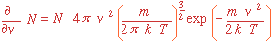# Review of Kinetic Theory

Molecules in an ideal gas have a Maxwellian velocity distribution:with mean velocity:Note in this formula you must use R in joules/kgm-mole!

The number of molecules impinging on a plane per square centimeter each second:Leading to a very important result: the maximum possible deposition flux for a given partial pressure of precursor, the

## Knudsen Equation:[where for convenience here P is in Torr, M in grams/mole, and J in molecules/cm2 second]

The mean free path of each molecule is:Notation:

N = number of moles (or molecules)

v = absolute value of velocity vector v

m = molecular mass

k = Boltzmann's constant, 1.38x10-23 J/K

R = universal gas constant

M = molar mass

n = molar volume N/V

a = effective molecular diameter